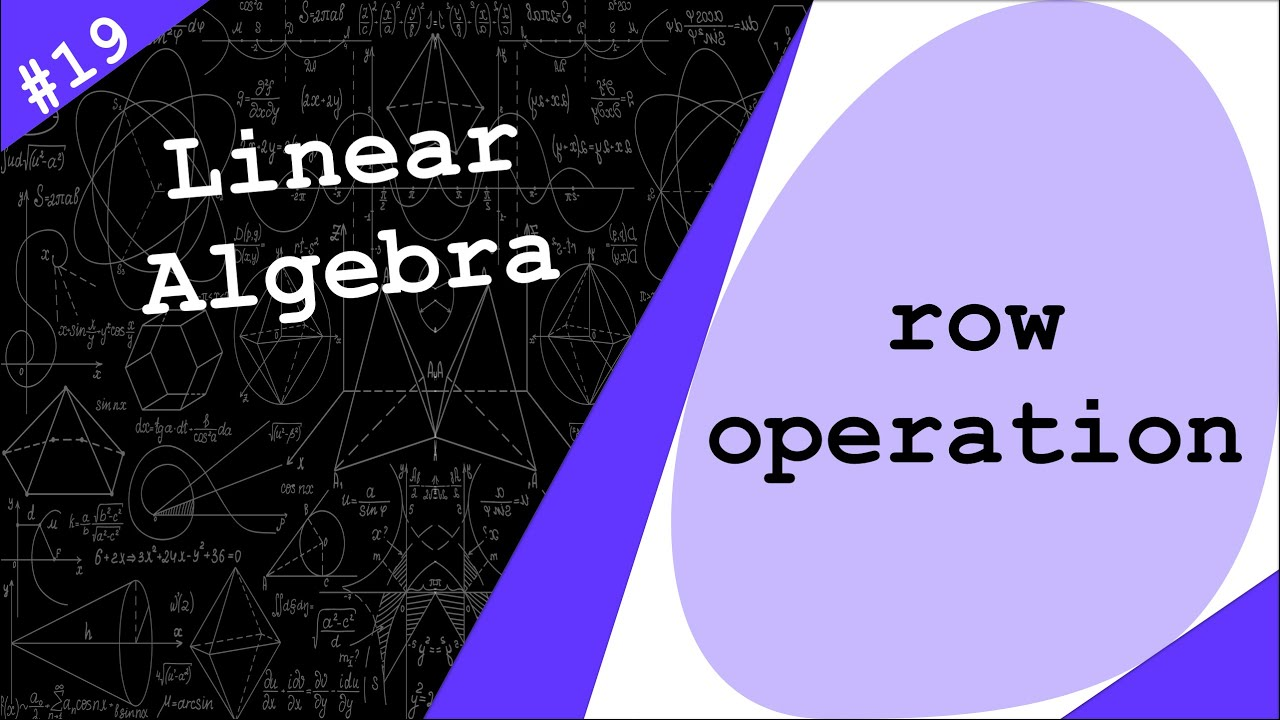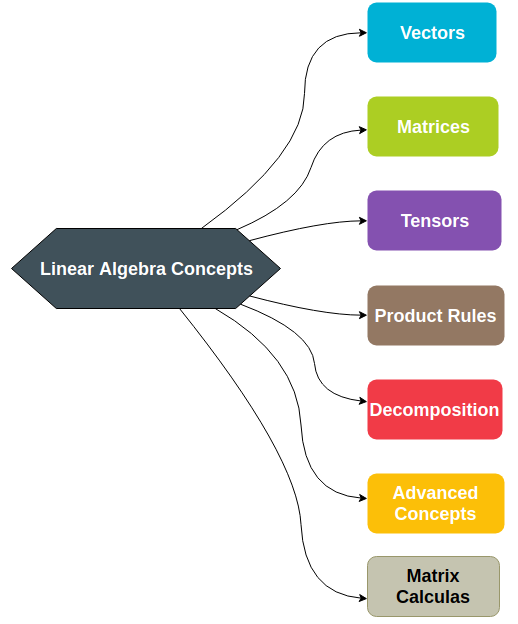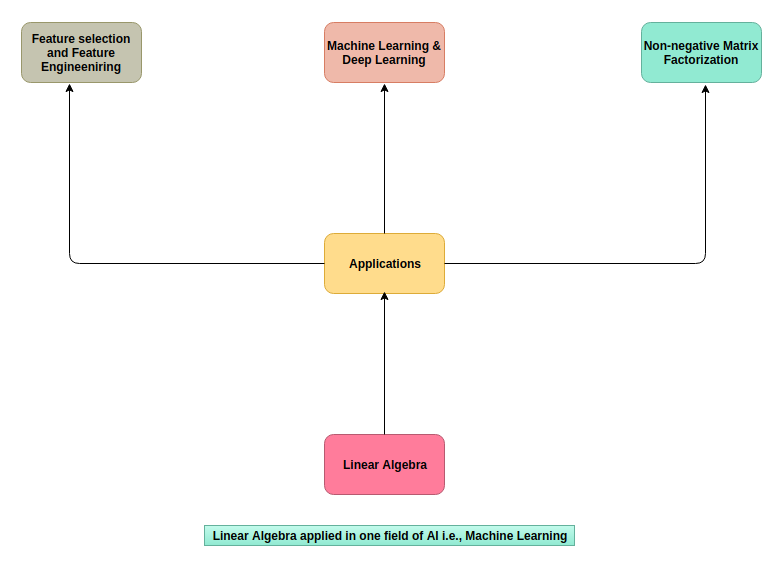1612971178

# An easy way to compute determinants | Linear Algebra - Dr. Ahmad Bazzi #19

Determinants could be computed by row operations. Indeed, determinants could be exhaustive to compute, but using row operations to obtain an equivalent triangular matrix makes determinant computation way easier. This lecture shows 4 properties that have to do with determinants when using row operations.

⏲Outline⏲

• 00:00 Introduction
• 00:30 Property 1: A determinant is a linear function of each row separately
• 02:35 Property 2: Zero determinant with two equal rows
• 03:01 Property 3: Flipping rows flips sign of determinant
• 03:58 Property 4: Unchanged determinant under row operations

#developer #programming

## Buddha Community1612971178

## An easy way to compute determinants | Linear Algebra - Dr. Ahmad Bazzi #19

Determinants could be computed by row operations. Indeed, determinants could be exhaustive to compute, but using row operations to obtain an equivalent triangular matrix makes determinant computation way easier. This lecture shows 4 properties that have to do with determinants when using row operations.

⏲Outline⏲

• 00:00 Introduction
• 00:30 Property 1: A determinant is a linear function of each row separately
• 02:35 Property 2: Zero determinant with two equal rows
• 03:01 Property 3: Flipping rows flips sign of determinant
• 03:58 Property 4: Unchanged determinant under row operations

#developer #programming1624447260

## Course Review: Python for Linear Algebra

Because I am continuously endeavouring to improve my knowledge and skill of the Python programming language, I decided to take some free courses in an attempt to improve upon my knowledge base. I found one such course on linear algebra, which I found on YouTube. I decided to watch the video and undertake the course work because it focused on the Python programming language, something that I wanted to improve my skill in. Youtube video this course review was taken from:- (4) Python for linear algebra (for absolute beginners) — YouTube

The course is for absolute beginners, which is good because I have never studied linear algebra and had no idea what the terms I would be working with were.

Linear algebra is the branch of mathematics concerning linear equations, such as linear maps and their representations in vector spaces and through matrices. Linear algebra is central to almost all areas of mathematics.

Whilst studying linear algebra, I have learned a few topics that I had not previously known. For example:-

A scalar is simply a number, being an integer or a float. Scalers are convenient in applications that don’t need to be concerned with all the ways that data can be represented in a computer.

A vector is a one dimensional array of numbers. The difference between a vector is that it is mutable, being known as dynamic arrays.

A matrix is similar to a two dimensional rectangular array of data stored in rows and columns. The data stored in the matrix can be strings, numbers, etcetera.

In addition to the basic components of linear algebra, being a scalar, vector and matrix, there are several ways the vectors and matrix can be manipulated to make it suitable for machine learning.

I used Google Colab to code the programming examples and the assignments that were given in the 1 hour 51 minute video. It took a while to get into writing the code of the various subjects that were studied because, as the video stated, it is a course for absolute beginners.

The two main libraries that were used for this course were numpy and matplotlib. Numpy is the library that is used to carry out algebraic operations and matplotlib is used to graphically plot the points that are created in the program.

#numpy #matplotlib #python #linear-algebra #course review: python for linear algebra #linear algebra1596273000

## Linear Algebra in Artificial Intelligence & Quantum Computing

Linear Algebra Usage Introduction: Linear Algebra is the primary computation tool in both Artificial Intelligence (AI) and **Quantum Computing (QC). **In this blog will cover the required topics for storage and computing for both the fields.Linear Algebra Concepts

Linear Algebra is basically a Mathematical Computational Tool and widely used in many areas like science , engineering and computational fields. Following diagram describes the concepts used in Linear Algebra for AI and Quantum Computing, first 4 topics (Vectors, Matrices, Tensors and Product Rules) covered in this article and the remaining topics are Decomposition ,**Advanced Concepts **for Quantum Computing, **Matrix Calculus **for Machine Learning and Deep Learning in other articles.

**Note: **Decomposition and Matrix Calculus links updated soon.

Primarily your data has to be stored in some where else to compute, Linear Algebra stores your data in Vectors, Matrices and Tensors and then operate as per your instructions. Linear Algebra is a Storage Space.

Title diagram shows what are mathematical objects as well as AI sub fields and Quantum Computing uses Linear Algebra.

Artificial Intelligence: Linear Algebra can be applied in Machine Learning and Artificial Intelligence, including exposure to clustering, data fitting, classification, validation, and feature engineering.

Linear algebra applied in unsupervised learning methods (like k-means) such as principal component analysis (PCA), non-negative matrix factorization and more sophisticated clustering methods. You can apply regression and classification methods , such as logistic regression and the support vector machine, as well as methods for computing model parameters that scale to extremely large scale problems.#artificial-intelligence #computer-science #linear-algebra #vector #quantum-computing1641805837

## How to Predict Housing Prices with Linear Regression?The final objective is to estimate the cost of a certain house in a Boston suburb. In 1970, the Boston Standard Metropolitan Statistical Area provided the information. To examine and modify the data, we will use several techniques such as data pre-processing and feature engineering. After that, we'll apply a statistical model like regression model to anticipate and monitor the real estate market.

Project Outline:

• EDA
• Feature Engineering
• Pick and Train a Model
• Interpret
• Conclusion

### EDA

Before using a statistical model, the EDA is a good step to go through in order to:

• Recognize the data set
• Check to see if any information is missing.
• Find some outliers.
• To get more out of the data, add, alter, or eliminate some features.

### Importing the Libraries

• Recognize the data set
• Check to see if any information is missing.
• Find some outliers.
• To get more out of the data, add, alter, or eliminate some features.

# Import the libraries #Dataframe/Numerical libraries import pandas as pd import numpy as np #Data visualization import plotly.express as px import matplotlib import matplotlib.pyplot as plt import seaborn as sns #Machine learning model from sklearn.linear_model import LinearRegression

### Have a Look at the Columns

Crime: It refers to a town's per capita crime rate.

ZN: It is the percentage of residential land allocated for 25,000 square feet.

Indus: The amount of non-retail business lands per town is referred to as the indus.

CHAS: CHAS denotes whether or not the land is surrounded by a river.

NOX: The NOX stands for nitric oxide content (part per 10m)

RM: The average number of rooms per home is referred to as RM.

AGE: The percentage of owner-occupied housing built before 1940 is referred to as AGE.

DIS: Weighted distance to five Boston employment centers are referred to as dis.

TAX: The TAX columns denote the rate of full-value property taxes per \$10,000 dollars.

B: B=1000(Bk — 0.63)2 is the outcome of the equation, where Bk is the proportion of blacks in each town.

PTRATIO: It refers to the student-to-teacher ratio in each community.

LSTAT: It refers to the population's lower socioeconomic status.

MEDV: It refers to the 1000-dollar median value of owner-occupied residences.

### Data Preprocessing

# Check if there is any missing values. housing_df.isna().sum() CRIM       0 ZN         0 INDUS      0 CHAS       0 NOX        0 RM         0 AGE        0 DIS        0 RAD        0 TAX        0 PTRATIO    0 B          0 LSTAT      0 MEDV       0 dtype: int64

No missing values are found

We examine our data's mean, standard deviation, and percentiles.

housing_df.describe()The crime, area, sector, nitric oxides, 'B' appear to have multiple outliers at first look because the minimum and maximum values are so far apart. In the Age columns, the mean and the Q2(50 percentile) do not match.

We might double-check it by examining the distribution of each column.

### Inferences

1. The rate of crime is rather low. The majority of values are in the range of 0 to 25. With a huge value and a value of zero.
2. The majority of residential land is zoned for less than 25,000 square feet. Land zones larger than 25,000 square feet represent a small portion of the dataset.
3. The percentage of non-retial commercial acres is mostly split between two ranges: 0-13 and 13-23.
4. The majority of the properties are bordered by the river, although a tiny portion of the data is not.
5. The content of nitrite dioxide has been trending lower from.3 to.7, with a little bump towards.8. It is permissible to leave a value in the range of 0.1–1.
6. The number of rooms tends to cluster around the average.
7. With time, the proportion of owner-occupied units rises.
8. As the number of weights grows, the weight distance between 5 employment centers reduces. It could indicate that individuals choose to live in new high-employment areas.
9. People choose to live in places with limited access to roadways (0-10). We have a 30th percentile outlier.
10. The majority of dwelling taxes are in the range of \$200-450, with large outliers around \$700,000.
11. The percentage of people with lower status tends to cluster around the median. The majority of persons are of lower social standing.

Because the model is overly generic, removing all outliers will underfit it. Keeping all outliers causes the model to overfit and become excessively accurate. The data's noise will be learned.

The approach is to establish a happy medium that prevents the model from becoming overly precise. When faced with a new set of data, however, they generalise well.

We'll keep numbers below 600 because there's a huge anomaly in the TAX column around 600.

new_df=housing_df[housing_df['TAX']<600]

### Looking at the DistributionThe overall distribution, particularly the TAX, PTRATIO, and RAD, has improved slightly.

### CorrelationPerfect correlation is denoted by the clear values. The medium correlation between the columns is represented by the reds, while the negative correlation is represented by the black.

With a value of 0.89, we can see that 'MEDV', which is the medium price we wish to anticipate, is substantially connected with the number of rooms 'RM'. The proportion of black people in area 'B' with a value of 0.19 is followed by the residential land 'ZN' with a value of 0.32 and the percentage of black people in area 'ZN' with a value of 0.32.

The metrics that are most connected with price will be plotted.## Feature Engineering

### Feature Scaling

Gradient descent is aided by feature scaling, which ensures that all features are on the same scale. It makes locating the local optimum much easier.

Mean standardization is one strategy to employ. It substitutes (target-mean) for the target to ensure that the feature has a mean of nearly zero.

def standard(X):    '''Standard makes the feature 'X' have a zero mean'''    mu=np.mean(X) #mean    std=np.std(X) #standard deviation    sta=(X-mu)/std # mean normalization    return mu,std,sta     mu,std,sta=standard(X) X=sta X

### Choose and Train the Model

For the sake of the project, we'll apply linear regression.

Typically, we run numerous models and select the best one based on a particular criterion.

Linear regression is a sort of supervised learning model in which the response is continuous, as it relates to machine learning.

Form of Linear Regression

y= θX+θ1 or y= θ1+X1θ2 +X2θ3 + X3θ4

y is the target you will be predicting

0 is the coefficient

x is the input

We will Sklearn to develop and train the model

#Import the libraries to train the model from sklearn.model_selection import train_test_split from sklearn.linear_model import LinearRegression

Allow us to utilise the train/test method to learn a part of the data on one set and predict using another set using the train/test approach.

X_train,X_test,y_train,y_test=train_test_split(X,y,test_size=0.4) #Create and Train the model model=LinearRegression().fit(X_train,y_train) #Generate prediction predictions_test=model.predict(X_test) #Compute loss to evaluate the model coefficient= model.coef_ intercept=model.intercept_ print(coefficient,intercept) [7.22218258] 24.66379606613584

In this example, you will learn the model using below hypothesis:

Price= 24.85 + 7.18* Room

It is interpreted as:

For a decided price of a house:

A 7.18-unit increase in the price is connected with a growth in the number of rooms.

As a side note, this is an association, not a cause!

### Interpretation

You will need a metric to determine whether our hypothesis was right. The RMSE approach will be used.

Root Means Square Error (RMSE) is defined as the square root of the mean of square error. The difference between the true and anticipated numbers called the error. It's popular because it can be expressed in y-units, which is the median price of a home in our scenario.

def rmse(predict,actual):    return np.sqrt(np.mean(np.square(predict - actual))) # Split the Data into train and test set X_train,X_test,y_train,y_test=train_test_split(X,y,test_size=0.4) #Create and Train the model model=LinearRegression().fit(X_train,y_train) #Generate prediction predictions_test=model.predict(X_test) #Compute loss to evaluate the model coefficient= model.coef_ intercept=model.intercept_ print(coefficient,intercept) loss=rmse(predictions_test,y_test) print('loss: ',loss) print(model.score(X_test,y_test)) #accuracy [7.43327725] 24.912055881970886 loss: 3.9673165450580714 0.7552661033654667 Loss will be 3.96

This means that y-units refer to the median value of occupied homes with 1000 dollars.

This will be less by 3960 dollars.

While learning the model you will have a high variance when you divide the data. Coefficient and intercept will vary. It's because when we utilized the train/test approach, we choose a set of data at random to place in either the train or test set. As a result, our theory will change each time the dataset is divided.

This problem can be solved using a technique called cross-validation.

### Improvisation in the Model

With 'Forward Selection,' we'll iterate through each parameter to assist us choose the numbers characteristics to include in our model.

### Forward Selection

1. Choose the most appropriate variable (in our case based on high correlation)
2. Add the next best variable to the model
3. Some predetermined conditions must meet.

We'll use a random state of 1 so that each iteration yields the same outcome.

cols=[] los=[] los_train=[] scor=[] i=0 while i < len(high_corr_var):    cols.append(high_corr_var[i])        # Select inputs variables    X=new_df[cols]        #mean normalization    mu,std,sta=standard(X)    X=sta        # Split the data into training and testing    X_train,X_test,y_train,y_test= train_test_split(X,y,random_state=1)        #fit the model to the training    lnreg=LinearRegression().fit(X_train,y_train)        #make prediction on the training test    prediction_train=lnreg.predict(X_train)        #make prediction on the testing test    prediction=lnreg.predict(X_test)        #compute the loss on train test    loss=rmse(prediction,y_test)    loss_train=rmse(prediction_train,y_train)    los_train.append(loss_train)    los.append(loss)        #compute the score    score=lnreg.score(X_test,y_test)    scor.append(score)        i+=1

We have a big 'loss' with a smaller collection of variables, yet our system will overgeneralize in this scenario. Although we have a reduced 'loss,' we have a large number of variables. However, if the model grows too precise, it may not generalize well to new data.

In order for our model to generalize well with another set of data, we might use 6 or 7 features. The characteristic chosen is descending based on how strong the price correlation is.

high_corr_var ['RM', 'ZN', 'B', 'CHAS', 'RAD', 'DIS', 'CRIM', 'NOX', 'AGE', 'TAX', 'INDUS', 'PTRATIO', 'LSTAT']

With 'RM' having a high price correlation and LSTAT having a negative price correlation.

# Create a list of features names feature_cols=['RM','ZN','B','CHAS','RAD','CRIM','DIS','NOX'] #Select inputs variables X=new_df[feature_cols] # Split the data into training and testing sets X_train,X_test,y_train,y_test= train_test_split(X,y, random_state=1) # feature engineering mu,std,sta=standard(X) X=sta # fit the model to the trainning data lnreg=LinearRegression().fit(X_train,y_train) # make prediction on the testing test prediction=lnreg.predict(X_test) # compute the loss loss=rmse(prediction,y_test) print('loss: ',loss) lnreg.score(X_test,y_test) loss: 3.212659865936143 0.8582338376696363

The test set yielded a loss of 3.21 and an accuracy of 85%.

Other factors, such as alpha, the learning rate at which our model learns, could still be tweaked to improve our model. Alternatively, return to the preprocessing section and working to increase the parameter distribution.

For more details regarding scraping real estate data you can contact Scraping Intelligence today

https://www.websitescraper.com/how-to-predict-housing-prices-with-linear-regression.php1602579600